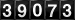## PROJECT: THE TOPOLOGY OF A/C MISSION SPACE

### Dr. Dean S. Hartley III

 Label Name Other Year DurationYrs Client JAD The Joint Staff Dates 1987 3 Employer DOE Oak Ridge Facilities Partner N/A Pubs Enhanced Utility of War Games Through AI lead author 1989
 Team Glenn O. Allgood, Mike A. Guth
 Artificial intelligence Expert systems Logistics issues Science, Math and Medicine Topology Transportation issues Warfare modeling

This project was part of the Modern Aids to Planning Program (MAPP), performed for The Joint Staff/JAD.

In defining an expert system for military air tasking orders , one of the authors (Allgood [external link]) defined a clustering system for defining the military roles of aircraft, based only on their physical characteristics. The concept of a cluster is not well defined mathematically, even though people have no difficulty communicating about them with each other. The evidence for the mathematical problem lies in the numerous different clustering algorithms and techniques being used on computers: if one algorithm were successful in identifying clusters within data that uniformly agreed with the human/visual decision, that one would be sufficient. Problems that humans can solve, but that resist computer solution, are almost by definition subjects for Artificial Intelligence study.

Initial examination of conceptual Aircraft (A/C) Mission Space identified three factors that held promise of discrimination between aircraft mission capabilities and measurement of extent of the capabilities: speed, weight, and width to length aspect ratio. As shown in Figure 1, project A/C space onto the speed cross weight plane, air superiority fighters have high speeds and medium weights; reciprocating (propeller-driven) attack airplanes have low weights and low speeds, etc.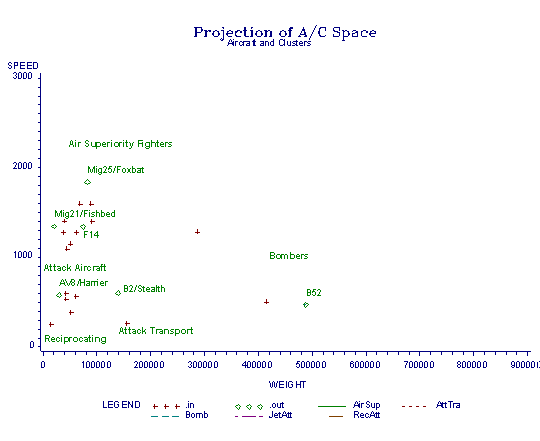Figure 1. Raw data

One central requirement for clusters is a distance function to determine how far from the cluster ideal any other aircraft is. There should be an ideal fighter aircraft, an ideal bomber, etc. For a given factor, the ideal might be an interior point or a boundary point. For instance, for mission X performed by a propeller aircraft, the ideal for the speed factor might be the fastest subsonic speed aircraft (propeller aircraft cannot exceed the speed of sound): a boundary point ideal. However, for mission Y for a jet, too little weight might be as bad as too much weight: an interior point ideal. Designing and building aircraft calls for compromises of many sorts, so that each aircraft departs from the ideal for each factor. It would be expected that a sufficiently large sample of aircraft would display a normal distribution about interior ideal points and half normal distributions about boundary ideal points. The distribution in A/C would then be fuzzy clusters, where all factors have interior ideals, and half or quarter fuzzy clusters, if one or two factors have boundary ideals.

Distance functions require topological spaces within which to be defined. Topological spaces with distances functions can be as tame as Euclidean 3-space, in which we live our everyday lives, or strange in ways only describable with complex mathematics. Some of these topological spaces can only be connected to Euclidean spaces in a piecewise manner, while others can be described as a transformation of Euclidean space by a single function. Some of the better behaved spaces have such functions with inverses, enabling the transformation of the space into the more congenial Euclidean space for analysis.

This examination concluded that the A/C space was not Euclidean, however not pathologically so. The space was transformed by taking the logarithm of two of the factors (speed and weight) and the ratio of the logarithms of the two components (width and length) of the third. The resulting transformed space, A/C', is Euclidean. Euclidean spaces will support more than one metric. A nearly trivial example of this is the ability to measure distances in feet and inches or in meters and centimeters and achieve consistent results, no matter what the use of the measurements. Notice that the standard practice is to measure length, width, and height all in the same units. This practice ensures that a diagonal measurement will have the same number attached to it to describe its length as will that same distance measured parallel to one of the axes of length, width, or height.

In neither A/C space, nor in A/C' space, is this common measurement system possible. In A/C space the units of measurement are weight on one axis, speed on another, and a ratio of lengths in the third. Natural units might be pounds or kilograms for one, miles per hour, knots, kilometers per hour, or Mach units (the speed of sound equals Mach 1) for the second, and dimensionless for the third. In the projection of A/C' space into its weight, speed subspace, the results of such choices are constant.

That is, a weight in kilograms is 2.2 times the weight in pounds. Its logarithm is equal to the logarithm of the weight in pounds plus the logarithm of 2.2. Equation 1 illustrates this.

Because log(a*z) = log(a)+log(z),

 distance = sqrt((log(a*x1)-log(a*x2))**2+(log(b*y1)-log(b*y2))**2) = sqrt((log(x1)-log(x2))**2+(log(y1)-log(y2))**2). (1)

Distances are not affected because the constant disappears in the distance calculation. This result does not obtain in the full (3-dimensional) A/C' space as shown in Equation 2.

 log(a*x1)/log(a*x2) = (log(a)+log(x1))/(log(a)+log(*x2)) not = log(x1)/log(x2) and not = log(a)+log(x1)/log(x2). (2)

This result means that the distance function in the full A/C' space is dependent upon the choices made for the units. This implication yields the necessary latitude for the second transformation discussed below.

A visual inspection of A/C' space (not shown in a figure) appears nearly ideal, the clusters defined by the algorithm match the human visual definition of clusters and appear ellipsoidal. A description of each ellipsoid requires a central point of three coordinates and a distance for each of the three axes of the ellipsoid, six numbers (there are more problems if the major axis of the ellipsoid is not oriented parallel to one of the dimensional axes). Given that the purpose of our original transformation of A/C space was to find a more congenial space in which to manipulate the data, a modification that improves the congeniality deserves consideration. In this case, if some transformation were possible that simultaneously converted each ellipsoid into a sphere, only four numbers would be required to describe each cluster: the three coordinates of the center and the radius. Fortuitously, such a transformation does exist. Multiplication of two of the axes by constants allows spherical definitions of the clusters. We will call the resulting space A/C".

Thus, A/C" has a metric with which we can measure the distance of any point to the center of any cluster. We can thus consider that any aircraft could be made to perform any mission; however, the greater the distance the less capable the aircraft would be expected to be in that role. Figure 2 shows the clustering of the aircraft in the projection of A/C" space onto the weight, speed subspace (actually log(weight) and log(speed)). Aircraft that fall outside of a cluster are shown with diamonds and labeled.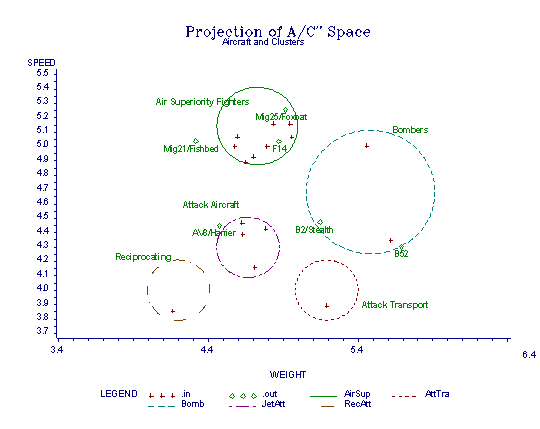Figure 2. Transformed data

Figure 3 shows the same data transformed to the corresponding projection of A/C space. The transformation of the circles indicates the nature of the non-Euclidean metric on A/C space.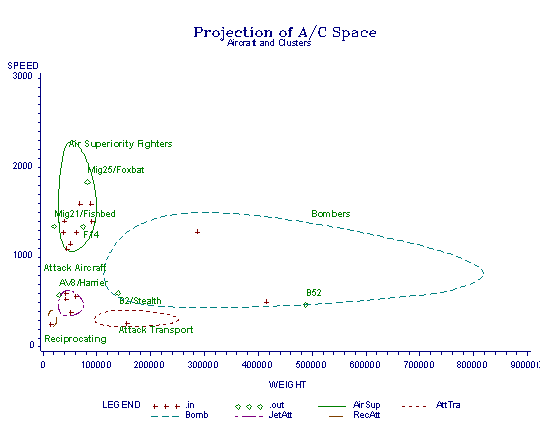Figure 3. Inverse transformed data with clusters

Both Fig. 3 and Fig. 2 display excellent separation of the aircraft into classifications, with the corresponding question as to the need for a third factor. The answer to this question has to do with the resulting explanatory power of the classification. That is, the AV8/Harrier is shown to be very close to the Jet Attack aircraft cluster, when it is known to be a good Air Superiority fighter in the right circumstances. Similarly, there are concerns with several of the aircraft labeled as outside clusters, but appearing to be inside.

Figure 4 shows the projection on the A/C" version of weight, aspect. The Harrier shows up as closer to the Air Superiority classification than to the Jet Attack classification in this projection. Some of the separations for other "outside" aircraft also become clear.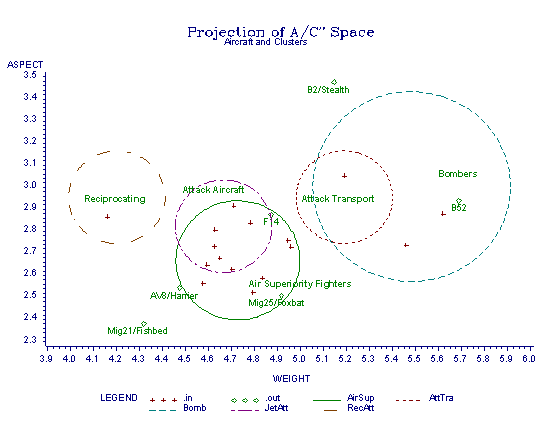Figure 4. Second projection of transformed data

Figure 5 show the projection on the A/C" version of speed, aspect. This figure shows the data from a third perspective. Notice that the Attack Transport and Reciprocating Attack clusters are superimposed. This indicates that the only difference in the definition of these two clusters is the location of the cluster centers in the weight dimension.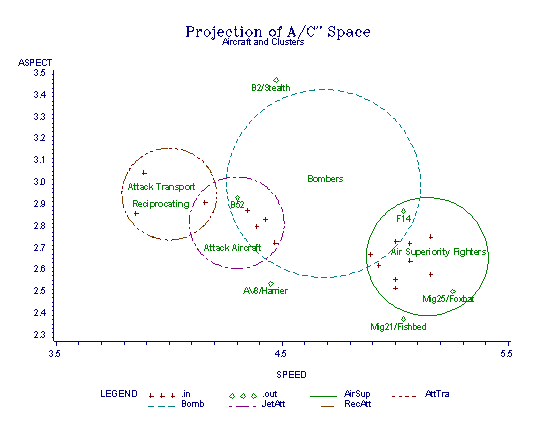Figure 5. Third projection of transformed data

Figure 6 shows a pseudo-3-dimensional view of A/C" space. Some of the "outside" aircraft and all of the "inside" aircraft are masked by the clusters. The cluster in the right foreground is the Reciprocating Attack cluster. The next lowest cluster is the Jet Attack cluster. The small, partially obscured cluster is the Attack Transport cluster (e.g., the AC-130). The medium-sized cluster in the center is the Air Superiority cluster. The largest cluster is the Bomber cluster.Figure 6. "3-D" view of transformed data

Because the classification algorithm was based on a sample of aircraft, some of the cluster radius values and center points are inexact. External aircraft characteristics were included in the final determinations to compensate for these problems. For instance, since (propeller driven) aircraft cannot exceed the speed of sound and have a practical lower limit to airspeed, these limits define the maximum radii for reciprocating aircraft.

 Hartley, D. S. III, G. O. Allgood, M. A. Guth. Enhanced Utility of War Games Through AI, ORNL/DSRD/TM-26. Martin Marietta, Oak Ridge, TN, 1988.

If you arrived here using a keyword shortcut, you may use your browser's "back" key to return to the keyword distribution page.